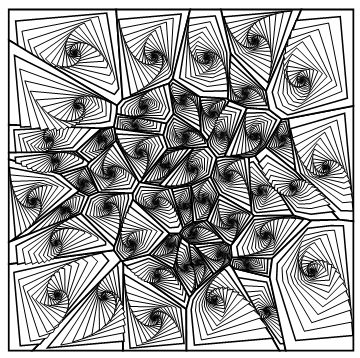图像的故事 – Zendoodle

• A+

介绍

Zendoodle是使用结构化图案或“Zeangle”绘制设计的艺术。

绘制方法

1. Clear[spiralize];
2. spiralize[p_, d_: 10, r_: 4, f_: 0.8, s_: 1, t_: 0.005] :=
3.  Module[{m, rr = r}, m = Mean@p[];
4.   Graphics[{EdgeForm[Thickness[t]], FaceForm[White],
5.     NestList[
6.      GeometricTransformation[
7.        GeometricTransformation[#,
8.         RotationTransform[rr++ s \[Degree], m]],
9.        ScalingTransform[{f, f}, m]] &, p, d]}]]
10. pts = RandomReal[{-1, 1}, {50, 2}];
11. polys = MeshPrimitives[VoronoiMesh[pts], 2];
12. Show[spiralize[#, 40, 5, 0.85] & /@ polys]• 微信公众号
• 关注微信公众号
•• QQ群
• 我们的QQ群号
•